Worksheet: Jugs and Mugs

# Worksheet: Jugs and Mugs - Notes | Study Mathematics for Class 2: NCERT - Class 2

 1 Crore+ students have signed up on EduRev. Have you?

Q.1. (A) Find the volume of each cylinder. (use π = 3.14)
(i)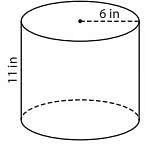Volume =____

(ii)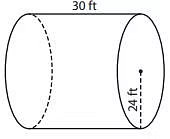Volume = ____

(iii)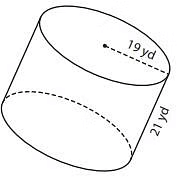Volume = ____

(B) Find the volume of each cylinder from the given parameters. (use π = 3.14)
(i) height = 9 ft ; radius = 3 ft
Volume = ____

(ii) radius = 13 yd ; height = 8 yd
Volume = ____

(iii) radius = 16 in ; height = 27 in
Volume = ____

(iv)  height = 15 ft ; radius = 10 ft
Volume = ____

(v) A cylindrical flower vase is 11-inch tall. Find the volume of the vase, if the radius is 4 inches. (use π = 3.14)

Q.2. (A) Find the volume of each triangular pyramid. Round your answer to two decimal places.
(i) Base Area = 40 ft2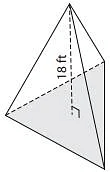Volume = ____

(ii) Base Area = 119 in2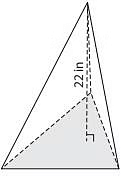Volume = ____
(iii) Base Area = 55 yd2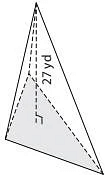Volume = ____

(iv) Base Area = 76 in2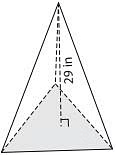Volume = ____

(v) Base Area = 23 yd2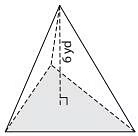Volume = ____

(vi) Base Area = 84 ft2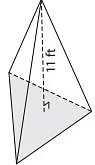Volume = ____

(B) The base area of a triangular pyramid is 65 square feet. Determine the volume, if its height is 24 feet.

(C) A triangular pyramid has a height of 14 inches and a base area of 94 square inches. Find the volume of the pyramid. Round your answer to two decimal places.

Q.3. (A) Find the indicated measure. Round your answer to the nearest tenth. (use π = 3.14)
(i) Volume = 157 ft3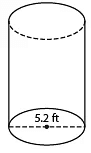height = ____

(ii) Volume = 910.6 yd3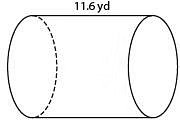diameter = ____

(iii) Volume = 9820.58 in3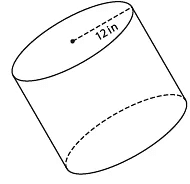height = ____

(B) Find the indicated measure. Round your answer to the nearest tenth. (use π = 3.14)
(i) Volume = 41,436 ft3 ; radius = 22.5 ft
height = ____

(ii) Volume = 68.13 yd3 ; height = 2.4 yd

(iii) Volume = 5,126.62 in3 ; height = 17 in
diameter = ____

(iv) Volume = 8,011.96 ft3 ; diameter = 30.2 ft
height = ____

(C) A cylindrical oil pipe measures 4 feet in diameter and 251.2 cubic feet in volume. What is the height of the oil pipe? Round your answer to the nearest tenth. (use π = 3.14)

The document Worksheet: Jugs and Mugs - Notes | Study Mathematics for Class 2: NCERT - Class 2 is a part of the Class 2 Course Mathematics for Class 2: NCERT.
All you need of Class 2 at this link: Class 2

## Mathematics for Class 2: NCERT

38 videos|62 docs|21 tests
 Use Code STAYHOME200 and get INR 200 additional OFF

## Mathematics for Class 2: NCERT

38 videos|62 docs|21 tests

Track your progress, build streaks, highlight & save important lessons and more!

,

,

,

,

,

,

,

,

,

,

,

,

,

,

,

,

,

,

,

,

,

;